# ILP Part 11 – Counting Sort

This is the eleventh part of the ILP series. For your convenience you can find other parts in the table of contents in Part 1 – Boolean algebra

Last time we implemented a selection sort in ILP. Today we are going to do something similar: we are going to implement counting sort.

# Introduction

As you probably remember from your classes, counting sort is useful when we try to sort values from small and finite set. It is for instance great for sorting people by their age because there are no people older than 200 years so we have at most two hundred possible values (and we can confine this range even more). What’s more, counting sort time complexity is linear so in theory it should work faster than selection sort. So let’s begin.

# Problem definition

First, let’s define necessary variables: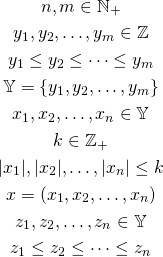We havevariables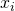to sort. Everyvariable has a value from set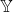. Sethas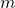variables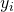. Variables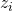represents the final result.
We are going to sort variablesusing the knowledge that they all come from the set.

# Algorithm

Our algorithm will have the following steps:

• Compare everywith every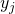• Sum results of comparisons to know how many values are there actually
• Construct the final vector

## Comparing

We define the following variables: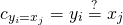We basically try to find them number of valuesin vector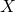. After this step we have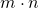different variables.

## Summing results

Now we need to know how many values are there so we define the following variables: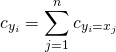Now we know that there are exactly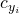valuesin vector. We now need to aggregate the results to be able to reconstruct the vector, so we define the partial sums: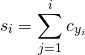This value tells us that there are exactly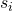elements not greater than. This might be a bit tricky so let’s consider an example first.

## Example

Let’s imagine that we want to sort the following vector: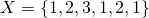We can see that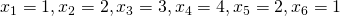and we have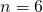values. We also assume the following set of possible values: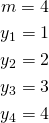Basically we assumed that our values are not greater than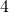and not less than. It is worth noting that inthere is no variables equal tobut this is how counting sort works. We now have the following results of comparisons: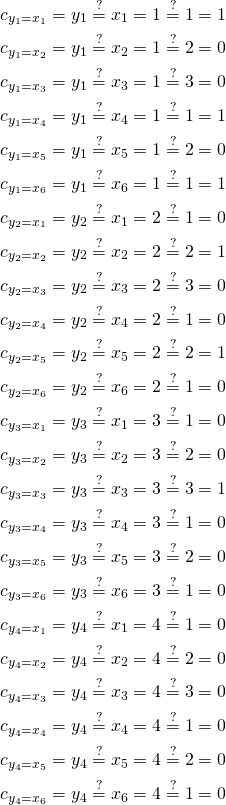Now we need to aggregate these values: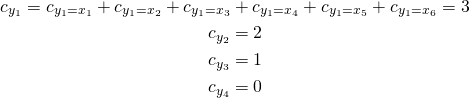Right now we know that there are exactlyvariables in vectorequal to. Now we sum the results: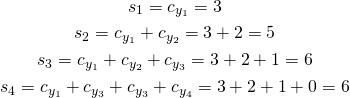And now we know that there are exactly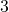values not greater than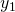in vector,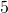values not greater than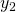and so on.

# Constructing the result

Every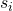variable tells us how many values not greater thanthere are in the original vector. We can utilize this knowledge to put values in correct places: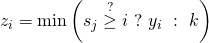In our example it is: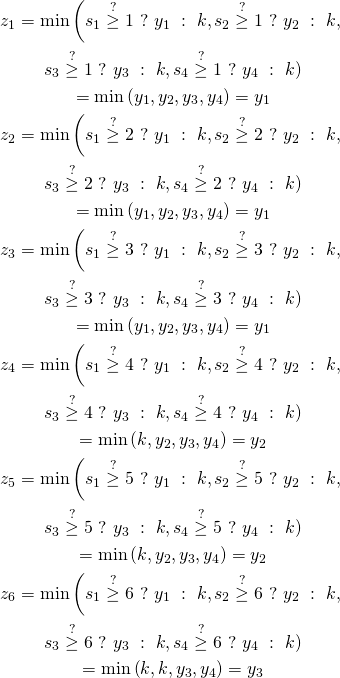This works in the following way: for every positionwe ask whether there are at leastelements not greater thanand we choose minimum ofvalues. So we basically try to insert values in their places and we need to usefunction to select lowest possible value for every place.

# Complexity

As you can see, this algorithm needstemporary variables. Assuming that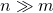our algorithm uses linear space. If it is not true thatthen it makes no sense using this algorithm but the same happens with casual counting sort. There is no use to sort numbers using this algorithm when the domain is really big.

# Summary

We already know two sorting algorithms. Their implementation is rather straightforward and really resembles the imperative counterparts. As an exercise you might want to implement other imperative algorithms using similar approach.